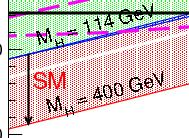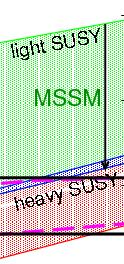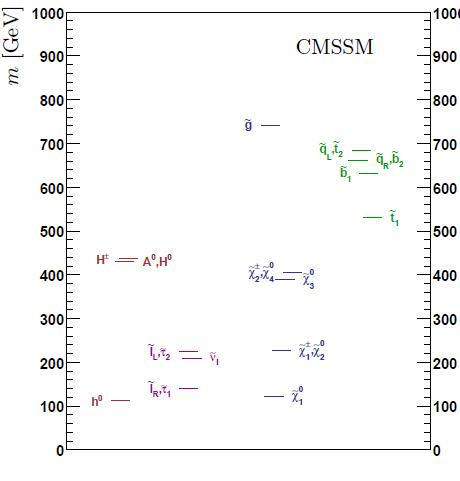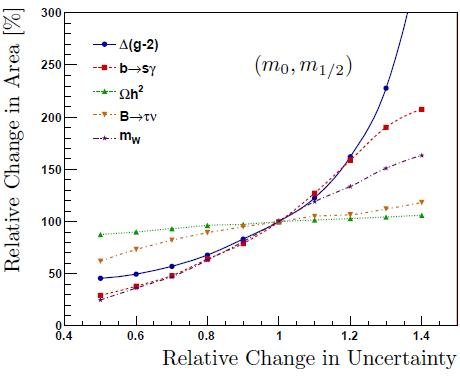## Zooming in on the HiggsMarch 24, 2009

Posted by dorigo in news, physics, science.
Tags: , , , , , , , , ,Yesterday Sven Heinemeyer kindly provided me with an updated version of a plot which best describes the experimental constraints on the Higgs boson mass, coming from electroweak observables measured at LEP and SLD, and from the most recent measurements of W boson and top quark masses. It is shown on the right (click to get the full-sized version).

The graph is a quite busy one, but I will try below to explain everything one bit at a time, hoping I keep things simple enough that a non-physicist can understand it.

The axes show suitable ranges of values of the top quark mass (varying on the horizontal axis) and of the W boson masses (on the vertical axis). The value of these quantities is functionally dependent (because of quantum effects connected to the propagation of the particles and their interaction with the Higgs field) on the Higgs boson mass.

The dependence, however, is really “soft”: if you were to double the Higgs mass by a factor of two from its true value, the effect on top and W masses would be only of the order of 1% or less. Because of that, only recently have the determinations of top quark and W boson masses started to provide meaningful inputs for a guess of the mass of the Higgs.

Top mass and W mass measurements are plotted in the graphs in the form of ellipses encompassing the most likely values: their size is such that the true masses should lie within their boundaries, 68% of the time. The red ellipse shows CDF results, and the blue one shows DZERO results.There is a third measurement of the W mass shown in the plot: it is displayed as a horizontal band limited by two black lines, and it comes from the LEP II measurements. The band also encompasses the 68% most likely W masses, as ellipses do.

In addition to W and top masses, other experimental results constrain the mass of top, W, and Higgs boson. The most stringent of these results are those coming from the LEP experiment at CERN, from detailed analysis of electroweak interactions studied in the production of Z bosons. A wide band crossing the graph from left to right, with a small tilt, encompasses the most likely region for top and W masses.So far we have described measurements. Then, there are two different physical models one should consider in order to link those measurements to the Higgs mass. The first one is the Standard Model: it dictates precisely the inter-dependence of all the parameters mentioned above. Because of the precise SM predictions, for any choice of the Higgs boson mass one can draw a curve in the top mass versus W mass plane. However, in the graph a full band is hatched instead. This correspond to allowing the Higgs boson mass to vary from a minimum of 114 GeV to 400 GeV. 114 GeV is the lower limit on the Higgs boson mass found by the LEP II experiments in their direct searches, using electron-positron collisions; while 400 GeV is just a reference value.

The boundaries of the red region show the functional dependence of Higgs mass on top and W masses: an increase of top mass, for fixed W mass, results in an increase of the Higgs mass, as is clear by starting from the 114 GeV upper boundary of the red region, since one then would move into the region, to higher Higgs masses. On the contrary, for a fixed top mass, an increase in W boson mass results in a decrease of the Higgs mass predicted by the Standard Model. Also note that the red region includes a narrow band which has been left white: it is the region corresponding to Higgs masses varying between 160 and 170 GeV, the masses that direct searches at the Tevatron have excluded at 95% confidence level.The second area, hatched in green, is not showing a single model predictions, but rather a range of values allowed by varying arbitrarily many of the parameters describing the supersymmetric extension of the SM called “MSSM”, its “minimal” extension. Even in the minimal extension there are about a hundred additional parameters introduced in the theory, and the values of a few of those modify the interconnection between top mass and W mass in a way that makes direct functional dependencies in the graph impossible to draw. Still, the hatched green region shows a “possible range of values” of the top quark and W boson masses. The arrow pointing down only describes what is expected for W and top masses if the mass of supersymmetric particles is increased from values barely above present exclusion limits to very high values.

So, to summarize, what to get from the plot ? I think the graph describes many things in one single package, and it is not easy to get the right message from it alone. Here is a short commentary, in bits.

• All experimental results are consistent with each other (but here, I should add, a result from NuTeV which finds indirectly the W mass from the measured ratio of neutral current and charged current neutrino interactions is not shown);
• Results point to a small patch of the plane, consistent with a light Higgs boson if the Standard Model holds
• The lower part of the MSSM allowed region is favored, pointing to heavy supersymmetric particles if that theory holds
• Among experimental determinations, the most constraining are those of the top mass; but once the top mass is known to within a few GeV, it is the W mass the one which tells us more about the unknown mass of the Higgs boson
• One point to note when comparing measurements from LEP II and the Tevatron experiments: when one draws a 2-D ellipse of 68% contour, this compares unfavourably to a band, which encompasses the same probability in a 1-D distribution. This is clear if one compares the actual measurements: CDF$80.413 \pm 48 MeV$ (with 200/pb of data), DZERO$80,401 \pm 44 MeV$ (with five times more statistics), LEP II$80.376 \pm 33 MeV$ (average of four experiments). The ellipses look like they are half as precise as the black band, while they are actually only 30-40% worse. If the above is obscure to you, a simple graphical explanation is provided here.
• When averaged, CDF and DZERO will actually beat the LEP II precision measurement -and they are sitting on 25 times more data (CDF) or 5 times more (DZERO).

## Predictions for SUSY particle masses!September 2, 2008

Posted by dorigo in cosmology, news, science.
Tags: , , , ,

Dear reader, if you are not a particle physicist you might find this post rather obscure. I apologize to you in that case, and I rather prefer to direct you to some easier discussion of Supersymmetry than attempting to shed light for you on the highly technical information discussed below:

• For an introduction, see here.
• For dark matter searches at colliders, see a three-part post here and here and here.
• Other dark matter searches and their implications for SUSY are discussed here.
• For a discussion of the status of Higgs searches and the implications of SUSY see here and here.
• For a discussion of the implications for supersymmetry of the g-2 measurement, see here;
• A more detailed discussion can be found in a report of a seminar by Massimo Passera on the topic, here and here.
• For$B \to \mu \mu$ searches and their impact on SUSY parameter space, see here.
• For other details on the subject, see this search result.
• And for past rumors on MSSM Higgs signals found at the Tevatron, have a look at these links.

If you have some background in particle physics, instead, you should definitely give a look at this new paper, appeared on August 29th in the arxiv. Like previous studies, it uses a wealth of experimental input coming from precision Standard Model electroweak observables, B physics measurements, and cosmological constraints to determine the allowed range of parameters within two constrained models of Supersymmetry -namely, the CMSSM and the NUHM1. However, the new study does much more than just turning a crank for you. Here is what you get in the package:

1. direct -and more up-to-date- assessments of the amount of data which LHC will need to wipe these models off the board, if they are incorrect;
2. a credible spectrum of the SUSY particle masses, for the parameters which provide the best agreement between experimental data and the two models considered;
3. a description of how much will be known about these models as soon as a few discoveries are made (if they are), such as the observation of an edge in the dilepton mass distribution extracted by CMS and ATLAS data;
4. a sizing up of the two models, CMSSM and NUHM1 -which are just special cases of the generic minimal supersymmetric extension of the standard model. Their relative merit in accommodating the current value of SM parameters is compared;
5. most crucially, a highly informative plot showing just how much we are going to learn on the allowed space of SUSY parameters from future improvements in a few important observables.

So, if you want to know what is currently the best estimate of the gluino mass: it is very high, above 700 GeV in the CMSSM and a bit below 600 for the NUHM1. The lightest Higgs boson, instead, is -perhaps unsurprisingly- lying very close to the lower LEP II limit, in the 115 GeV ballpark (actually, even a bit lower than that, but that is a detail – read the paper if you want to know more about that). The LSP is instead firmly in the 100 GeV range. For instance, check the figure below, showing the best fit for the CMSSM (which, by the way, implies$M_0 = 60 GeV$,$M_{1/2}=310 GeV$,$A_0 = 240 GeV$, and$\tan \beta =11$).The best plots are however the two I attach below: they represent a commendable effort to make things simpler for us. Really a highly distilled result of the gazillions of CPU-intensive computations which went into the determination of the area of parameter space that current particle physics measurements are allowing. In them, you can read out the relative merit of future improvements in a few of the most crucial measurements in electroweak physics, B physics, and cosmology, as far as our knowledge of MSSM parameters are concerned. The allowed area in the space of two parameters –$m_0 \div m_{1/2}$ as well as$m_0 \div \tan \beta$, at 95% confidence level, is studied as a function of the variation in the total uncertainty on five quantities: the error on the gyromagnetic ratio of the muon,$\Delta (g-2)_\mu$, the uncertainty in the radiative decay$b \to s \gamma$, the uncertainty in cold dark matter$\Omega h^2$, the branching fraction of$B \to \tau \nu$ decays, and the W boson mass$M_W$.Extremely interesting stuff! one learns that future improvements in the measurement of the dark matter fraction will yield NO improvement in the constraints of the MSSM parameter space. In a way, dark matter does point to a sparticle candidate, but WMAP has already measured it too well!

Another point to make from the graphs above is that of the observables listed the W boson mass is the one whose uncertainty is going to be reduced sizably very soon -that is where we expect to be improving matters most in the near future, of course if LHC does not see SUSY before! Instead, the$b \to s \gamma$ branching fraction uncertainty might actually turn out to need larger uncertainties than those assumed in the paper, making the allowed MSSM parameter space larger rather than smaller. As for the muon$g-2$, things can go in both directions there as well, as more detailed estimates of the current uncertainties are revised. These issues are discussed in detail in the paper, so I have better direct you to it rather than inserting my own misunderstandings.

Finally, the current fits slightly favor the NUHM1 scenario (the single-parameter Non-Universal Higgs Model) over the CMSSM. The NUHM1 scenario includes one further parameter, governing the difference between the soft SUSY-breaking contribution to$M_H^2$ and to squark and sleptons masses. The overall best-fit$\chi^2$ is better, and this implies that the additional parameter is used successfully by the fitter. The lightest Higgs boson mass also comes up at a “non-excluded” value of 118 GeV, higher than for the best fit point of the CMSSM.